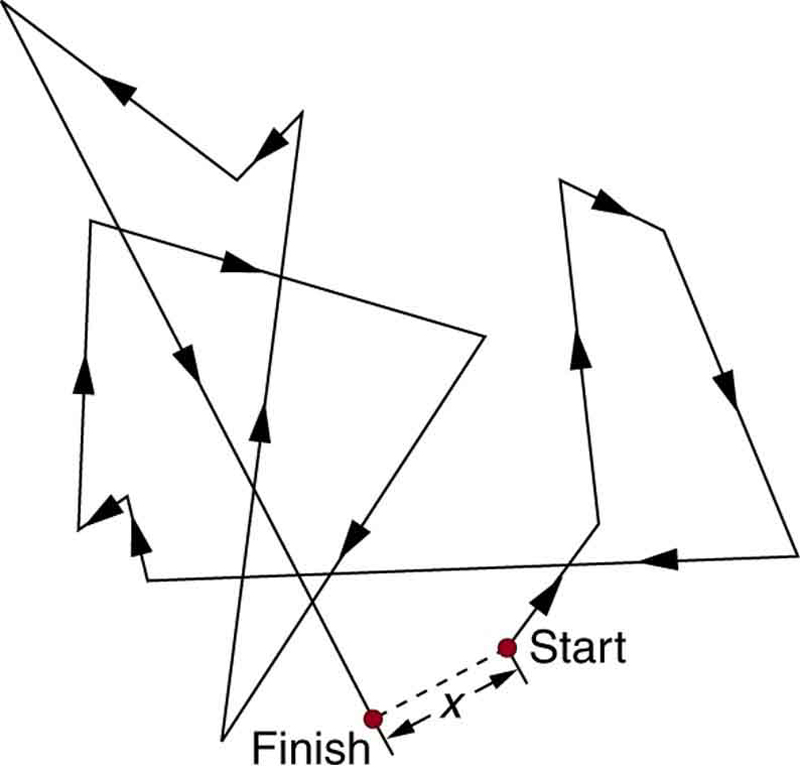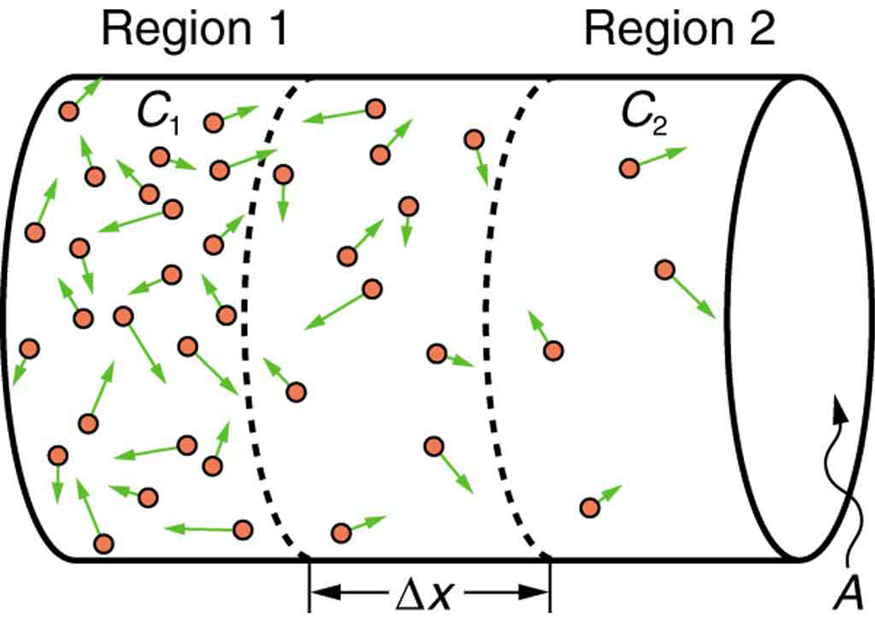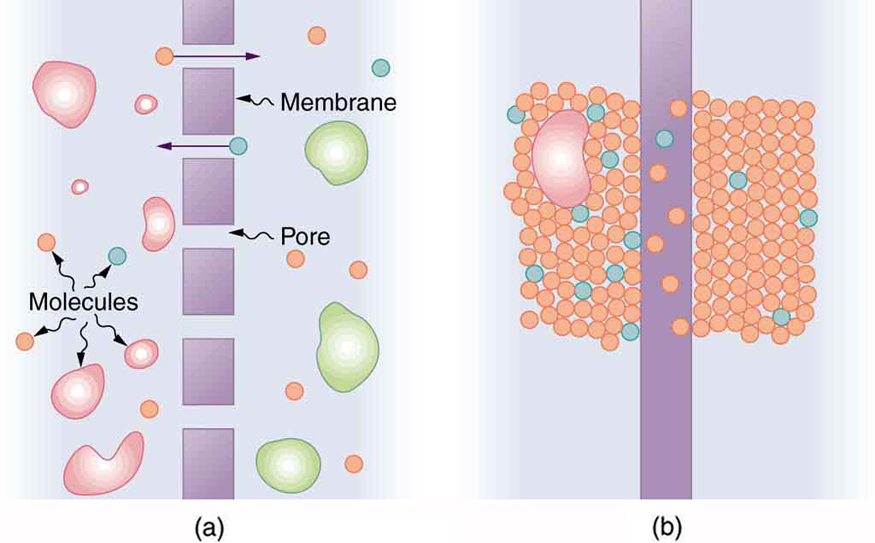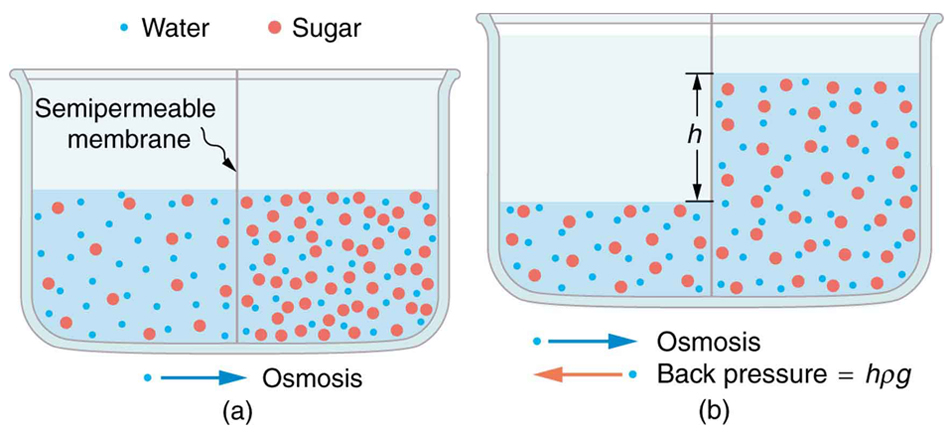Chapter 12 Fluid Dynamics and Its Biological and Medical Applications

# 91 12.7 Molecular Transport Phenomena: Diffusion, Osmosis, and Related Processes

### Summary

• Define diffusion, osmosis, dialysis, and active transport.
• Calculate diffusion rates.

# Diffusion

There is something fishy about the ice cube from your freezer—how did it pick up those food odors? How does soaking a sprained ankle in Epsom salt reduce swelling? The answer to these questions are related to atomic and molecular transport phenomena—another mode of fluid motion. Atoms and molecules are in constant motion at any temperature. In fluids they move about randomly even in the absence of macroscopic flow. This motion is called a random walk and is illustrated in Figure 1. Diffusion is the movement of substances due to random thermal molecular motion. Fluids, like fish fumes or odors entering ice cubes, can even diffuse through solids.

Diffusion is a slow process over macroscopic distances. The densities of common materials are great enough that molecules cannot travel very far before having a collision that can scatter them in any direction, including straight backward. It can be shown that the average distance$\boldsymbol{x_{\textbf{rms}}}$that a molecule travels is proportional to the square root of time:

$\boldsymbol{x_{\textbf{rms}}\:=\sqrt{2Dt}},$

where$\boldsymbol{x_{\textbf{rms}}}$stands for the root-mean-square distance and is the statistical average for the process. The quantity$\boldsymbol{D}$is the diffusion constant for the particular molecule in a specific medium. Table 2 lists representative values of$\boldsymbol{D}$for various substances, in units of$\boldsymbol{\textbf{m}^2\textbf{/s}}.$Figure 1. The random thermal motion of a molecule in a fluid in time t. This type of motion is called a random walk.
Diffusing molecule Medium D (m2/s)
Hydrogen$\boldsymbol{(\textbf{H}_2)}$ Air $\boldsymbol{6.4\times10^{-5}}$
Oxygen$\boldsymbol{(\textbf{O}_2)}$ Air $\boldsymbol{1.8\times10^{-5}}$
Oxygen$\boldsymbol{(\textbf{O}_2)}$ Water $\boldsymbol{1.0\times10^{-9}}$
Glucose$\boldsymbol{(\textbf{C}_6\textbf{H}_{12}\textbf{O}_6)}$ Water $\boldsymbol{6.7\times10^{-10}}$
Hemoglobin Water $\boldsymbol{6.9\times10^{-11}}$
DNA Water $\boldsymbol{1.3\times10^{-12}}$
Table 2. Diffusion Constants for Various Molecules1

Note that$\boldsymbol{D}$gets progressively smaller for more massive molecules. This decrease is because the average molecular speed at a given temperature is inversely proportional to molecular mass. Thus the more massive molecules diffuse more slowly. Another interesting point is that$\boldsymbol{D}$for oxygen in air is much greater than$\boldsymbol{D}$for oxygen in water. In water, an oxygen molecule makes many more collisions in its random walk and is slowed considerably. In water, an oxygen molecule moves only about$\boldsymbol{40\:\mu\textbf{m}}$in 1 s. (Each molecule actually collides about$\boldsymbol{10^{10}}$times per second!). Finally, note that diffusion constants increase with temperature, because average molecular speed increases with temperature. This is because the average kinetic energy of molecules,$\boldsymbol{\frac{1}{2}mv^2},$is proportional to absolute temperature.

### Example 1: Calculating Diffusion: How Long Does Glucose Diffusion Take?

Calculate the average time it takes a glucose molecule to move 1.0 cm in water.

Strategy

We can use$\boldsymbol{x_{\textbf{rms}}=\sqrt{2Dt}},$the expression for the average distance moved in time$\boldsymbol{t},$and solve it for$\boldsymbol{t}.$All other quantities are known.

Solution

Solving for$\boldsymbol{t}$and substituting known values yields

$\begin{array}{lcl} \boldsymbol{t} & \boldsymbol{=} & \boldsymbol{\frac{x_{\textbf{rms}}^2}{2D}=\frac{(0.010\textbf{ m})^2}{2(6.7\times10^{-10}\textbf{ m}^2\textbf{/s})}} \\ {} & \boldsymbol{=} & \boldsymbol{7.5\times10^4\textbf{ s}=21\textbf{ h.}} \end{array}$

Discussion

This is a remarkably long time for glucose to move a mere centimeter! For this reason, we stir sugar into water rather than waiting for it to diffuse.

Because diffusion is typically very slow, its most important effects occur over small distances. For example, the cornea of the eye gets most of its oxygen by diffusion through the thin tear layer covering it.

# The Rate and Direction of Diffusion

If you very carefully place a drop of food coloring in a still glass of water, it will slowly diffuse into the colorless surroundings until its concentration is the same everywhere. This type of diffusion is called free diffusion, because there are no barriers inhibiting it. Let us examine its direction and rate. Molecular motion is random in direction, and so simple chance dictates that more molecules will move out of a region of high concentration than into it. The net rate of diffusion is higher initially than after the process is partially completed. (See Figure 2.)Figure 2. Diffusion proceeds from a region of higher concentration to a lower one. The net rate of movement is proportional to the difference in concentration.

The rate of diffusion is proportional to the concentration difference. Many more molecules will leave a region of high concentration than will enter it from a region of low concentration. In fact, if the concentrations were the same, there would be no net movement. The rate of diffusion is also proportional to the diffusion constant$\boldsymbol{D},$which is determined experimentally. The farther a molecule can diffuse in a given time, the more likely it is to leave the region of high concentration. Many of the factors that affect the rate are hidden in the diffusion constant$\boldsymbol{D}.$For example, temperature and cohesive and adhesive forces all affect values of$\boldsymbol{D}.$

Diffusion is the dominant mechanism by which the exchange of nutrients and waste products occur between the blood and tissue, and between air and blood in the lungs. In the evolutionary process, as organisms became larger, they needed quicker methods of transportation than net diffusion, because of the larger distances involved in the transport, leading to the development of circulatory systems. Less sophisticated, single-celled organisms still rely totally on diffusion for the removal of waste products and the uptake of nutrients.

# Osmosis and Dialysis—Diffusion across Membranes

Some of the most interesting examples of diffusion occur through barriers that affect the rates of diffusion. For example, when you soak a swollen ankle in Epsom salt, water diffuses through your skin. Many substances regularly move through cell membranes; oxygen moves in, carbon dioxide moves out, nutrients go in, and wastes go out, for example. Because membranes are thin structures (typically$\boldsymbol{6.5\times10^{-9}}$to$\boldsymbol{10\times10^{-9}}$m across) diffusion rates through them can be high. Diffusion through membranes is an important method of transport.

Membranes are generally selectively permeable, or semipermeable. (See Figure 3.) One type of semipermeable membrane has small pores that allow only small molecules to pass through. In other types of membranes, the molecules may actually dissolve in the membrane or react with molecules in the membrane while moving across. Membrane function, in fact, is the subject of much current research, involving not only physiology but also chemistry and physics.Figure 3. (a) A semipermeable membrane with small pores that allow only small molecules to pass through. (b) Certain molecules dissolve in this membrane and diffuse across it.

Osmosis is the transport of water through a semipermeable membrane from a region of high concentration to a region of low concentration. Osmosis is driven by the imbalance in water concentration. For example, water is more concentrated in your body than in Epsom salt. When you soak a swollen ankle in Epsom salt, the water moves out of your body into the lower-concentration region in the salt. Similarly, dialysis is the transport of any other molecule through a semipermeable membrane due to its concentration difference. Both osmosis and dialysis are used by the kidneys to cleanse the blood.

Osmosis can create a substantial pressure. Consider what happens if osmosis continues for some time, as illustrated in Figure 4. Water moves by osmosis from the left into the region on the right, where it is less concentrated, causing the solution on the right to rise. This movement will continue until the pressure$\boldsymbol{\rho{gh}}$created by the extra height of fluid on the right is large enough to stop further osmosis. This pressure is called a back pressure. The back pressure$\boldsymbol{\rho{g}h}$that stops osmosis is also called the relative osmotic pressure if neither solution is pure water, and it is called the osmotic pressure if one solution is pure water. Osmotic pressure can be large, depending on the size of the concentration difference. For example, if pure water and sea water are separated by a semipermeable membrane that passes no salt, osmotic pressure will be 25.9 atm. This value means that water will diffuse through the membrane until the salt water surface rises 268 m above the pure-water surface! One example of pressure created by osmosis is turgor in plants (many wilt when too dry). Turgor describes the condition of a plant in which the fluid in a cell exerts a pressure against the cell wall. This pressure gives the plant support. Dialysis can similarly cause substantial pressures.Figure 4. (a) Two sugar-water solutions of different concentrations, separated by a semipermeable membrane that passes water but not sugar. Osmosis will be to the right, since water is less concentrated there. (b) The fluid level rises until the back pressure ρgh equals the relative osmotic pressure; then, the net transfer of water is zero.

Reverse osmosis and reverse dialysis (also called filtration) are processes that occur when back pressure is sufficient to reverse the normal direction of substances through membranes. Back pressure can be created naturally as on the right side of Figure 4. (A piston can also create this pressure.) Reverse osmosis can be used to desalinate water by simply forcing it through a membrane that will not pass salt. Similarly, reverse dialysis can be used to filter out any substance that a given membrane will not pass.

One further example of the movement of substances through membranes deserves mention. We sometimes find that substances pass in the direction opposite to what we expect. Cypress tree roots, for example, extract pure water from salt water, although osmosis would move it in the opposite direction. This is not reverse osmosis, because there is no back pressure to cause it. What is happening is called active transport, a process in which a living membrane expends energy to move substances across it. Many living membranes move water and other substances by active transport. The kidneys, for example, not only use osmosis and dialysis—they also employ significant active transport to move substances into and out of blood. In fact, it is estimated that at least 25% of the body’s energy is expended on active transport of substances at the cellular level. The study of active transport carries us into the realms of microbiology, biophysics, and biochemistry and it is a fascinating application of the laws of nature to living structures.

# Section Summary

• Diffusion is the movement of substances due to random thermal molecular motion.
• The average distance$\boldsymbol{x_{\textbf{rms}}}$a molecule travels by diffusion in a given amount of time is given by
$\boldsymbol{x_{\textbf{rms}}=\sqrt{2Dt},}$

where$\boldsymbol{D}$is the diffusion constant, representative values of which are found in Table 2.

• Osmosis is the transport of water through a semipermeable membrane from a region of high concentration to a region of low concentration.
• Dialysis is the transport of any other molecule through a semipermeable membrane due to its concentration difference.
• Both processes can be reversed by back pressure.
• Active transport is a process in which a living membrane expends energy to move substances across it.

### Conceptual Questions

1: Why would you expect the rate of diffusion to increase with temperature? Can you give an example, such as the fact that you can dissolve sugar more rapidly in hot water?

2: How are osmosis and dialysis similar? How do they differ?

### Problems & Exercises

1: You can smell perfume very shortly after opening the bottle. To show that it is not reaching your nose by diffusion, calculate the average distance a perfume molecule moves in one second in air, given its diffusion constant$\boldsymbol{D}$to be$\boldsymbol{1.00\times10^{-6}\textbf{ m}^2\textbf{/s}}.$

2: What is the ratio of the average distances that oxygen will diffuse in a given time in air and water? Why is this distance less in water (equivalently, why is$\boldsymbol{D}$less in water)?

3: Oxygen reaches the veinless cornea of the eye by diffusing through its tear layer, which is 0.500-mm thick. How long does it take the average oxygen molecule to do this?

4: (a) Find the average time required for an oxygen molecule to diffuse through a 0.200-mm-thick tear layer on the cornea. (b) How much time is required to diffuse$\boldsymbol{0.500\textbf{ cm}^3}$of oxygen to the cornea if its surface area is$\boldsymbol{1.00\textbf{ cm}^2}?$

5: Suppose hydrogen and oxygen are diffusing through air. A small amount of each is released simultaneously. How much time passes before the hydrogen is 1.00 s ahead of the oxygen? Such differences in arrival times are used as an analytical tool in gas chromatography.

## Footnotes

1. 1 At 20°C and 1 atm

## Glossary

diffusion
the movement of substances due to random thermal molecular motion
semipermeable
a type of membrane that allows only certain small molecules to pass through
osmosis
the transport of water through a semipermeable membrane from a region of high concentration to one of low concentration
dialysis
the transport of any molecule other than water through a semipermeable membrane from a region of high concentration to one of low concentration
relative osmotic pressure
the back pressure which stops the osmotic process if neither solution is pure water
osmotic pressure
the back pressure which stops the osmotic process if one solution is pure water
reverse osmosis
the process that occurs when back pressure is sufficient to reverse the normal direction of osmosis through membranes
reverse dialysis
the process that occurs when back pressure is sufficient to reverse the normal direction of dialysis through membranes
active transport
the process in which a living membrane expends energy to move substances across

### Solutions

Problems & Exercises

1:

$\boldsymbol{1.41\times10^{-3}\textbf{ m}}$

3:

$\boldsymbol{1.3\times10^2\textbf{ s}}$

5:

0.391 s